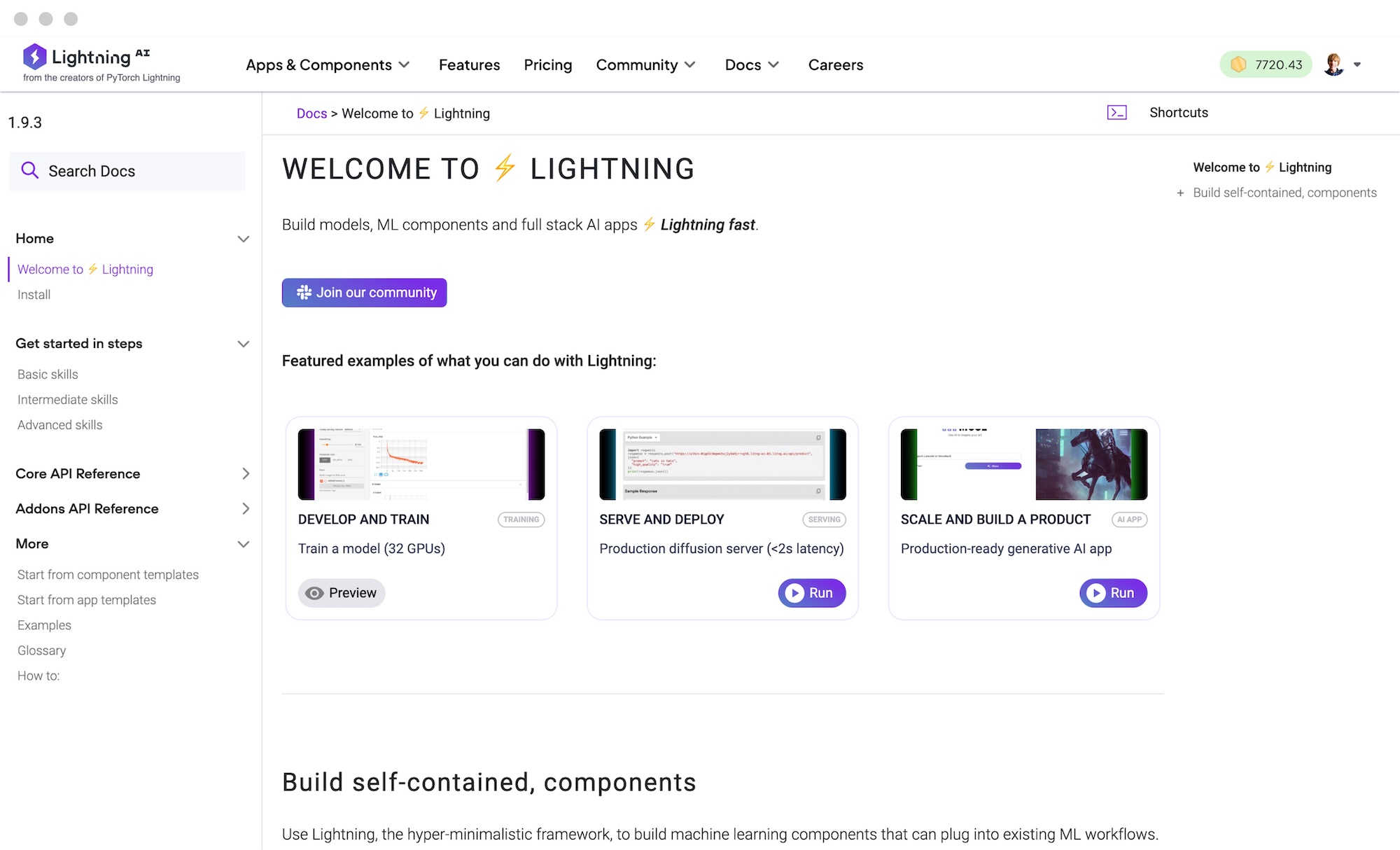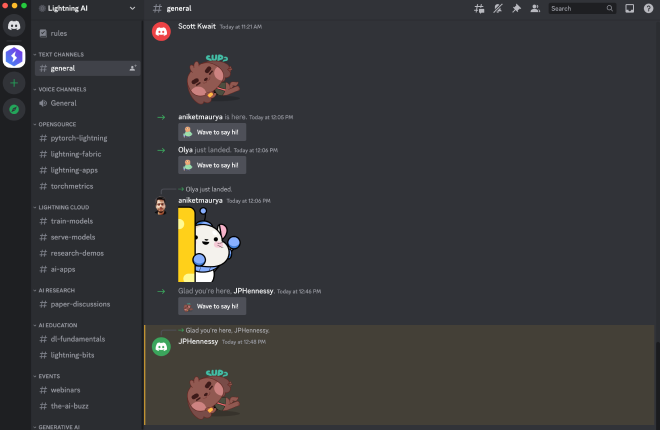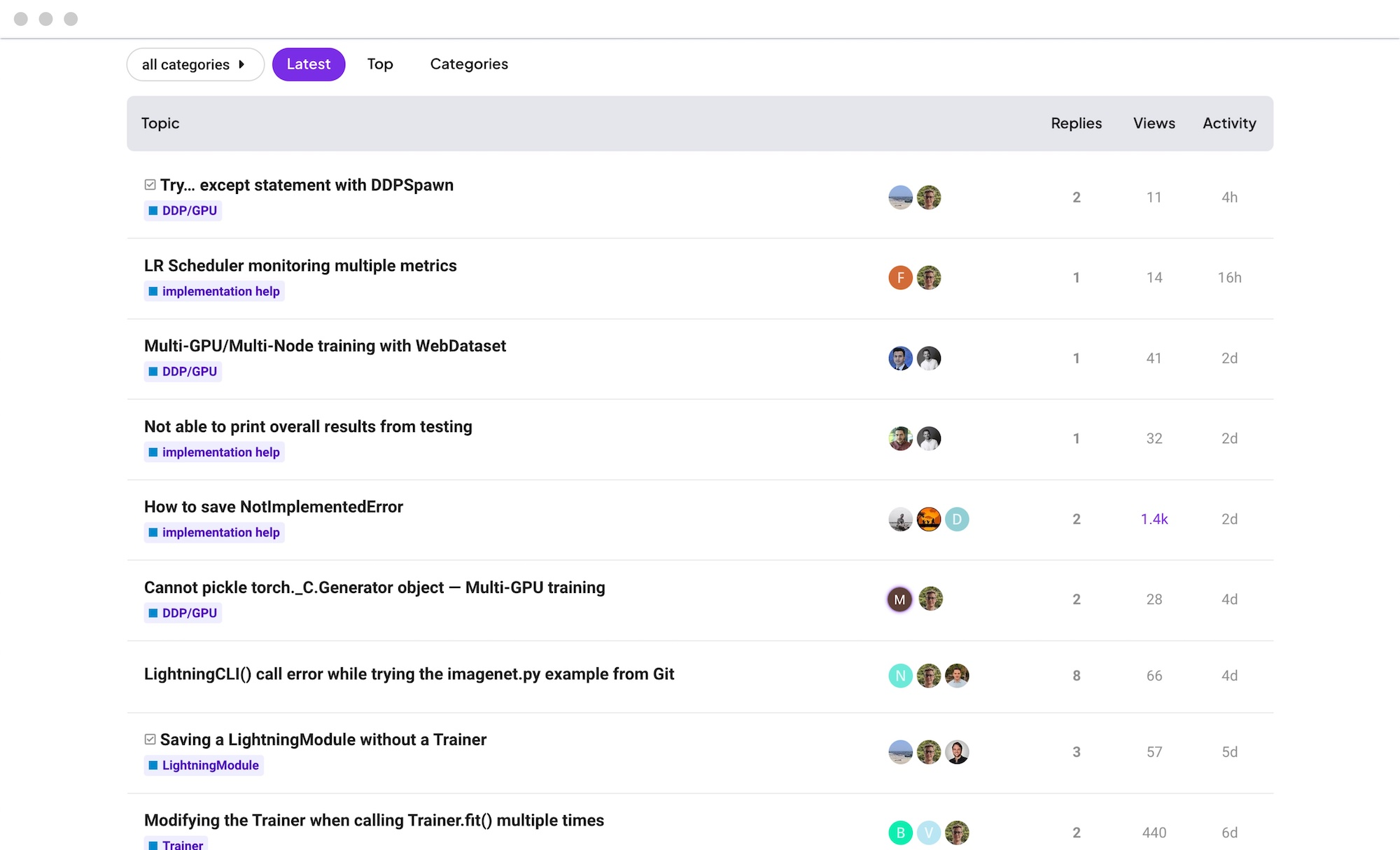Introducing Lit-LLaMA: a minimal, optimized rewrite of LLaMA licensed under Apache 2.0 →

# Scale your models, without the boilerplate

Lightning’s open-source ecosystem is designed for researchers and developers who require flexibility and performance at scale.### Build AI without the boilerplate

Lightning simplifies your deep learning code by taking care of engineering boilerplate, so you can focus on the problems that matter to you.### Unlock deep learning at scale

Work seamlessly with distributed computing environments like multi-GPU and TPU clusters and scale projects to large models and data.### Create with the community

Join over 100,000 users and companies using Lightning to create their AI future. Tap into cutting-edge research and take it to production.

###PyTorch Lightning

PyTorch Lightning structures your deep learning code and manages your training loop, unlocking productivity and scale at the flip of a switch.

This framework is for researchers and ML practitioners who want to build models that are easy to write, run, scale, read, and debug.

``````
import lightning as L
from torch import nn, optim, utils
import torchvision

encoder = nn.Sequential(nn.Linear(28 * 28, 64), nn.ReLU(), nn.Linear(64, 3))
decoder = nn.Sequential(nn.Linear(3, 64), nn.ReLU(), nn.Linear(64, 28 * 28))

class LitAutoEncoder(L.LightningModule):
def __init__(self, encoder, decoder):
super().__init__()
self.encoder = encoder
self.decoder = decoder

def training_step(self, batch, batch_idx):
x, y = batch
x = x.view(x.size(0), -1)
z = self.encoder(x)
x_hat = self.decoder(z)
loss = nn.functional.mse_loss(x_hat, x)
self.log("train_loss", loss)
return loss

def configure_optimizers(self):
return optimizer

autoencoder = LitAutoEncoder(encoder, decoder)

# setup data

# train the model
trainer = L.Trainer(max_epochs=2)
``````
``````
import lightning as L
from torch import nn, optim, utils
import torchvision

def main():
# create models
encoder = nn.Sequential(nn.Linear(28 * 28, 64), nn.ReLU(), nn.Linear(64, 3))
decoder = nn.Sequential(nn.Linear(3, 64), nn.ReLU(), nn.Linear(64, 28 * 28))

params = list(encoder.parameters()) + list(decoder.parameters())

# setup data

# setup Fabric
fabric = L.Fabric()
encoder, optimizer = fabric.setup(encoder, optimizer)
decoder = fabric.setup(decoder)

# train the model
for epoch in range(2):
fabric.print("Epoch:", epoch)
# get the inputs; data is a list of [inputs, labels]
x, y = batch
x = x.view(x.size(0), -1)

# forward + loss
z = encoder(x)
x_hat = decoder(z)
loss = nn.functional.mse_loss(x_hat, x)

# backward + optimize
fabric.backward(loss)
optimizer.step()

if i % 100 == 0:
fabric.print("train_loss", float(loss))

fabric.log("train_loss", loss)

if __name__ == "__main__":
main()
``````

###Lightning Fabric New!

Lightning Fabric gives you full control over your training loop and allows you to leverage tools like callbacks and checkpoints only when needed.

Use this library for complex tasks like reinforcement learning, active learning, and transformers without losing control over your training code.

## The Lightning Continuum##Docs

Glossary, tutorials, and code samples to guide you as you build with Lightning.##Discord

Ask questions, give feedback, and help define the direction of Lightning.##AI Education

Learn deep learning with a modern open source stack.##Forums

Start a discussion or browse our archives of tips and best practices.